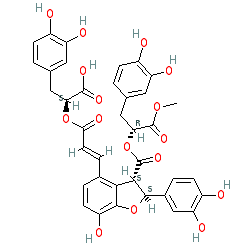=====================================================SMILES: O=C(O)[C@@H](OC(/C=C/C1=C2[C@H](C(O[C@@H](C(OC)=O)CC3=CC(O)=C(O)C=C3)=O)[C@@H](C4=CC(O)=C(O)C=C4)OC2=C(O)C=C1)=O)CC5=CC(O)=C(O)C=C5
InChIKey: REHAMWBRZKUHPN-RTLLXKGUSA-N

–EOF–